# PRACTICAL APPROACH ON WORKING WITH LIST IN PYTHON PROGRAMMING

in STEMGeeks6 months agoImage Source

In one of my previous post about list, I have explained about the concepts of list along with creating a list, modifying an item in a list like updating, deleting, looping through a list and finally sorting a list. In this post, I am mainly focusing on an important concept of list in python i.e. Slicing. Slicing means creating a new list from existing list by specifying certain conditions. Along with slicing, I will be doing other great cool things with list like creating a new list from empty list, finding minimum and maximum values in a list and the use of in-built function called `range()` to create a list.

Slicing a list is easier to do and is just a one line of code. To slice a list, we need to specify the index number of the first element and the last element that you want it to be in a new list. Lets see with the following code:

``````list1 = ["Valerie", "John", "Anon", "Zoe", "Alice", "Ryan", "Donnie", "Brock", "Karen", "Carl", "Sean"]

new_list = list1[0:5]

print(new_list)
``````

Here, we have a list of person name and suppose we want to create a new list from Valerie to Alice. For this we specify the initial index number as 0 as we want first element to be Valerie and the last index number as 5. So we will write it as `list1[0:5]`. It will print up to Alice, which is index number 4 and this won't actually print value in index number 5. If you want to print value in index 5 then you should slice the list as `list1[0:6]`. I hope you grasp the concept. Now lets see the actual output:

Now if you want to create a new list from Anon (index 2) to Brock (index 7), then you will slice the list with this syntax `list1[2:8]`. You will see the output as below: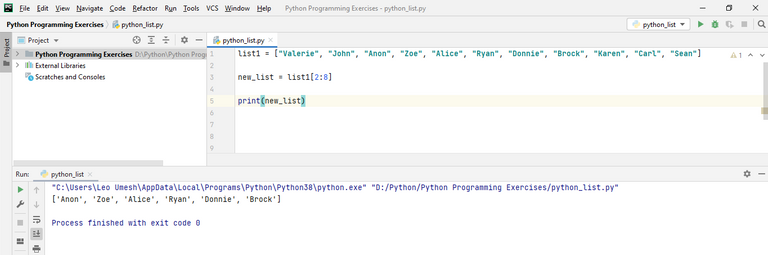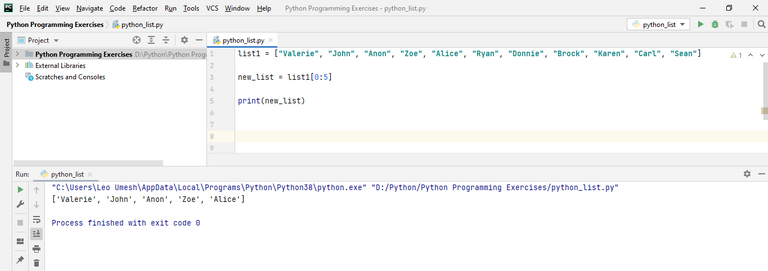If you forget to write the initial index number like `list1[:3]`, then it will slice from the first item in the list. Lets try testing this out in our IDE.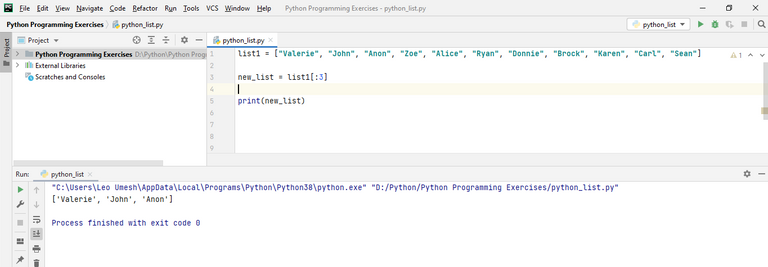Similarly if you want to slice a list starting from Zoe (index number 3) to the end, you can use `list1[3:]`.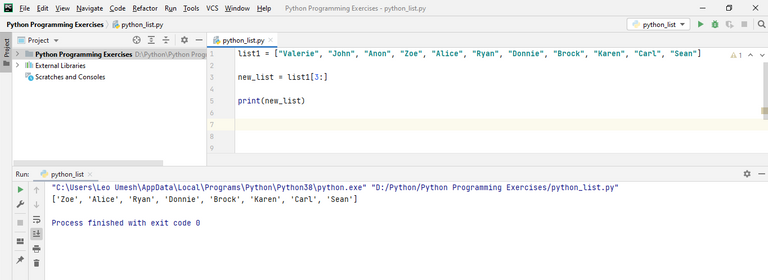You can use a negative number to slice the list also. Using negative number will slice the list from the end of our original list. For example, lets try to print out this statement `list1[-1:]`.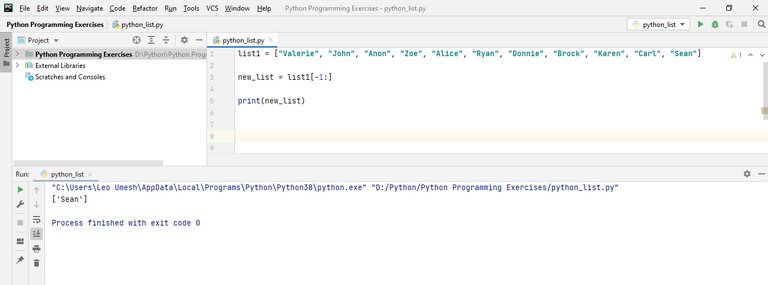You can see here a new list is being created where the first element is the last element of our original list. Lets suppose we want to create a new list from Sean (index number -1) to Brock (index number -4). For this we will slice with this syntax `list1[-4:]`. Lets see the output here: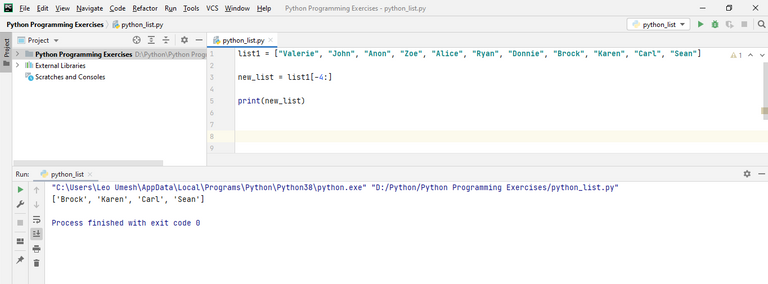That was all about slicing in the list. Now lets see about creating a nested list. It means a list which again contains a list. Lets suppose we have list1 containing names and list2 containing numerical values. We want to create another list i.e. list3 that contains a list of list1 and list2. We will write following code for it:

``````list1 = ["Valerie", "John", "Anon", "Zoe", "Alice", "Ryan", "Donnie", "Brock", "Karen", "Carl", "Sean"]

list2 = [12, 13, 56, 65, 23, 90, 22, 102, 78]

list3 = [list1, list2]

print(list3)
``````

If you run this program, it will give following output: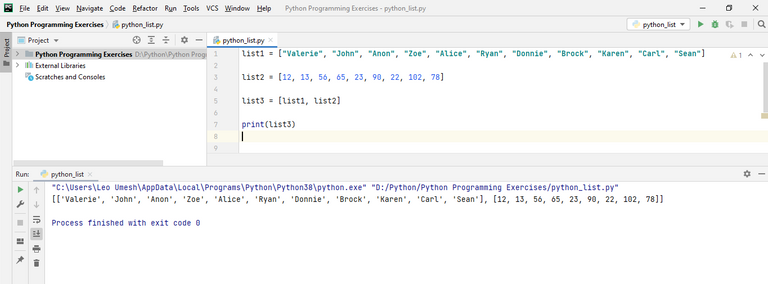In the above code, lets try to find maximum and minimum digits of list2 which is 12 and 102 respectively. We will use python in built `min()` and `max()` function.

``````list2 = [12, 13, 56, 65, 23, 90, 22, 102, 78]

print(min(list2))

print(max(list2))
``````

Running this code will give you following output.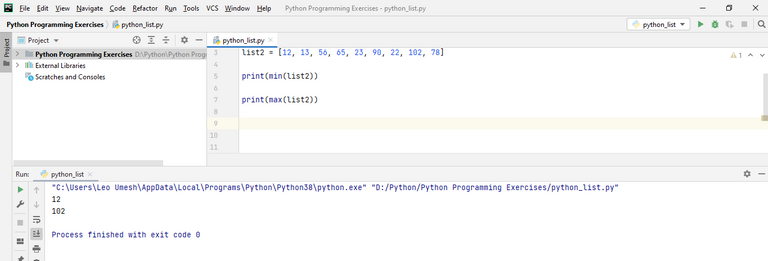Lets suppose we have this another list of small numbers. We want to find the sum of all the items in the list. For this one, we use `sum()` method.

``````list4 = [2, 6, 8, 3, 5]

print(sum(list4))

``````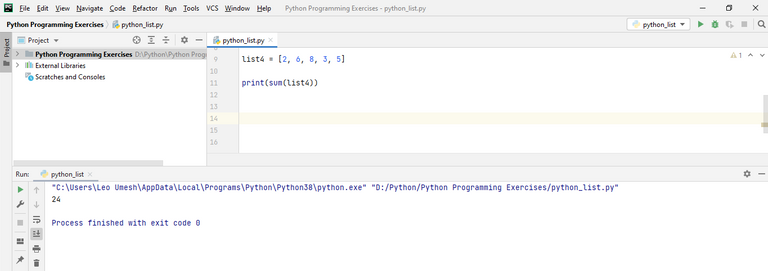At last, we will see the use of `range()` function to create a new list. Lets suppose we want to create a list of number from 1 to 5. For this we will define a range function: `range(1,6)`. Also note down here that this will create a number from 1 to 5 and not from 1 to 6. Then we want to wrap this in a list using `list()` function. See the below code along with demo.

``````list5 = list(range(1,6))

print(list5)
``````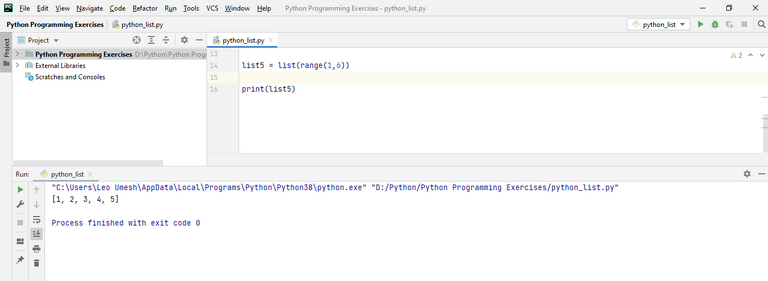You can see that our desired list has been created. Also we can create a list of odd number. For this we should specify extra parameter in the `range()` function at the end. In the below code we have used `list(range(1,17,2))`. This will create a list where the first number is 1. As last parameter of this function is 2, it will add 2 to 1 which is 3 and at the next iteration it will again add 2 to 3 which is 5 and again it will add 2 to 5 which is 7 and so on unless the last value reaches 17. But remember it won't print last value itself as we discussed above.

``````list5 = list(range(1,17,2))

print(list5)
``````

The output will be odd number from 1 to 16 as below: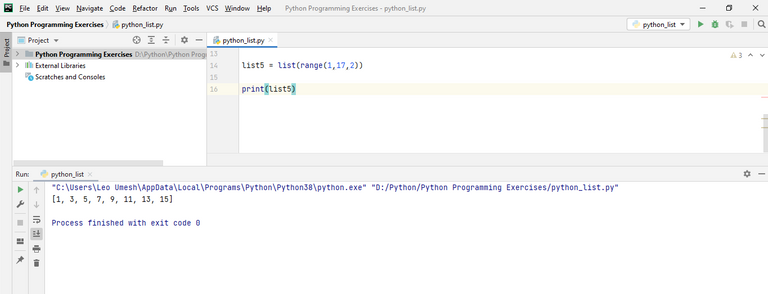You can try checking all above code online here.